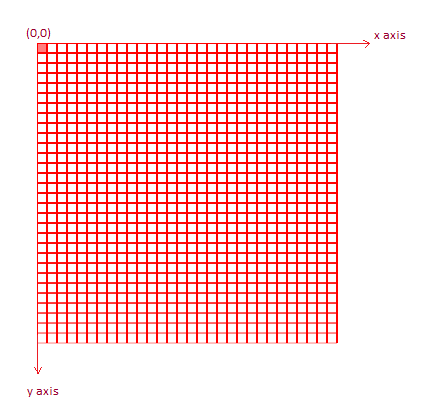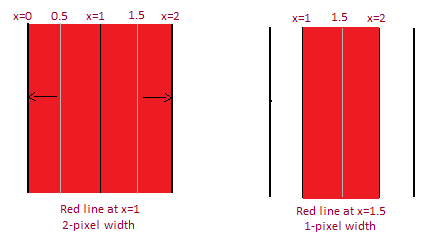Introduction to HTML5 Canvas Element  The canvas element is basically a space on a HTML page that can be used for graphics and animation. You can define the width of this space using the 'width' and 'height' attributes. By default, the width and height will be 300 and 150 pixels respectively (According to current HTML5 standard) The basics of drawing on canvas The canvas is a 2-D array of pixels having an x-axis and y-axis. The origin of the canvas is at the top left corner. Every point on the canvas can be represented by an x-coordinate and y-coordinate. Every point that you draw on the canvas is 1 pixel in length and breath.Assuming that the grid above is a canvas area, each box on the grid is a pixel. The first pixel (filled in red) will have coordinates x=0 and y = 0. While drawing lines on the canvas, the width of the line may vary slightly from what you specify using the lineWidth() attribute. In the below example, the black lines form the vertical boundary for a pixel. If you try to draw a 1-pixel line from (1,0) to (1,10), then the width of the line will fall 0.5 pixels on either side of x = 1. However, since half a pixel can not be drawn on, the width of the line will be extended (rounded up) so that it now becomes a 2-pixel line going from x = 0 to x = 2. On the other hand, if you draw a 1-pixel line from (1.5,0) to (1.5,10), the width of the line will fall 0.5 pixels on either side of x= 1.5. You will now et a 1-pixel line from (1,0) to (2,0).